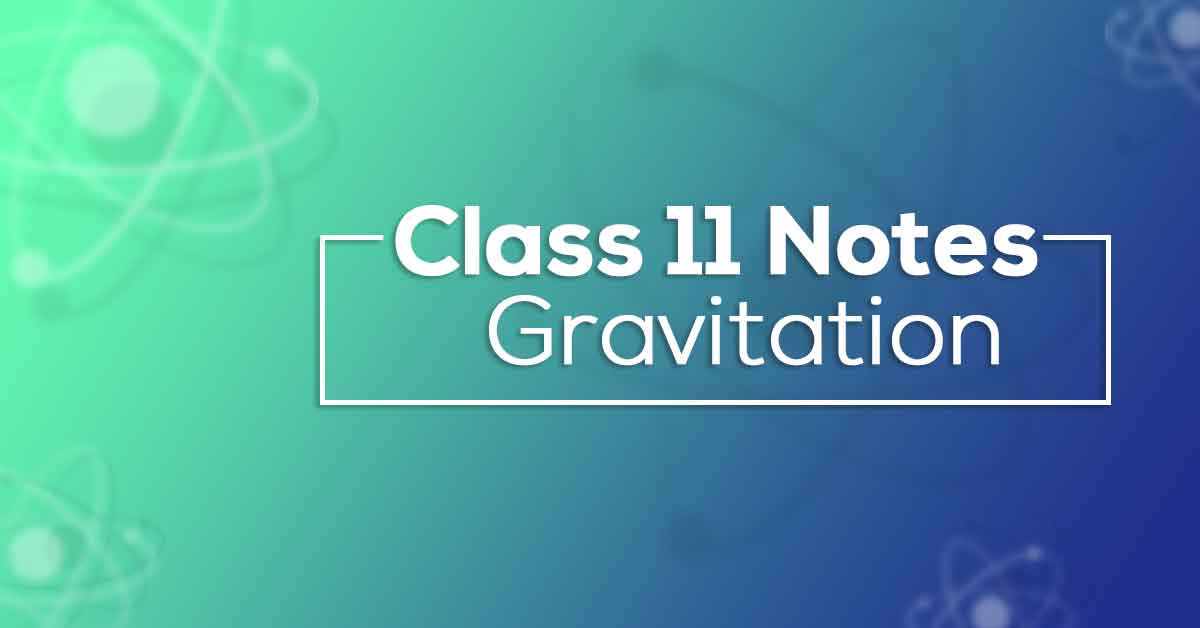Class 11 Physics Chapter 8 Gravitation Notes | Vidyakul
×

# Class 11 Physics Chapter 8 Gravitation NotesClass 11 Physics Chapter 8 Gravitation Notes aims at increasing the self-confidence of the students by offering a simple way to study or revise the chapter.  These notes provide the students with the summary of the chapter, important points to remember and a detailed explanation of important concepts and derivations for better understanding. Gravitation Class 11 Notes aims would fuel your exam preparation which ultimately leads you to score maximum marks in Exams.

### Definition Of Gravitation.

Newton was the first scientist who studied the force of gravitation. According to him there is a force which is exerted by the surface of the earth because of which all the objects are attracted towards the surface of the earth. He also concluded that all objects in this universe attract each other with a force. This force is Gravitation force. If the product of mass of the bodies increase the force of attraction also increases between them and if the square of the distance between the bodies increases, force decreases.

#### Know more about the same in Class 11 Physics Chapter 8 Gravitation Notes pdf.